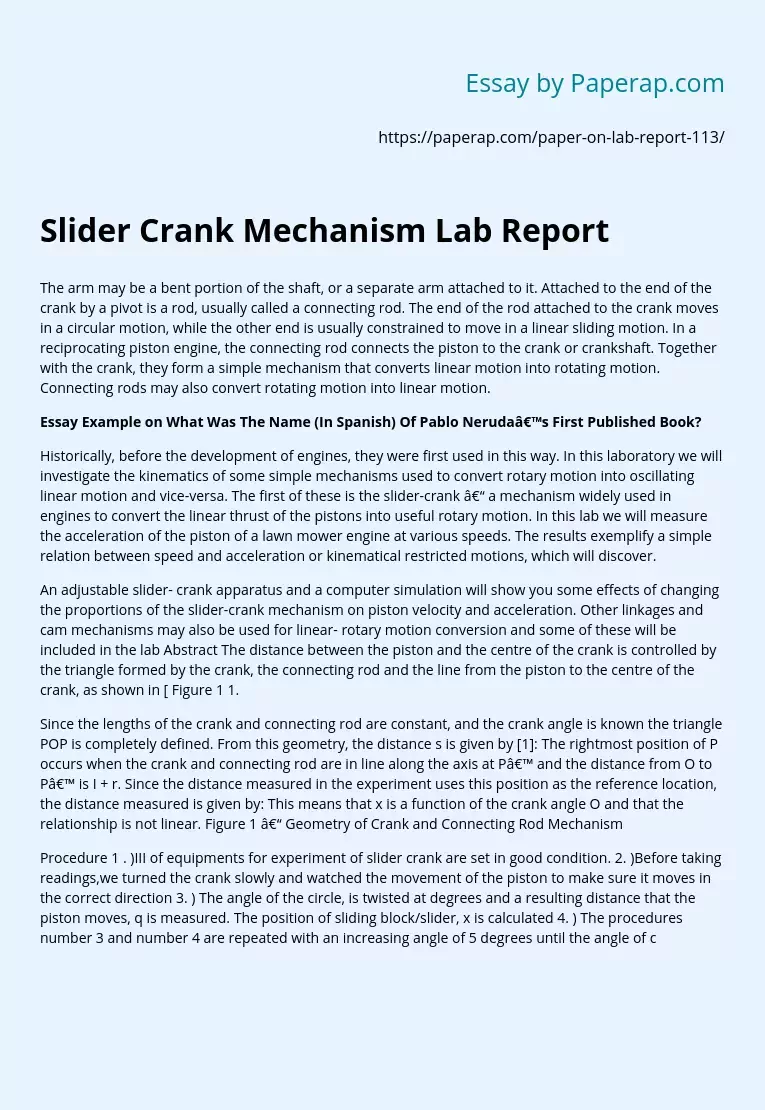# Slider Crank Mechanism Lab Report

The arm may be a bent portion of the shaft, or a separate arm attached to it. Attached to the end of the crank by a pivot is a rod, usually called a connecting rod. The end of the rod attached to the crank moves in a circular motion, while the other end is usually constrained to move in a linear sliding motion. In a reciprocating piston engine, the connecting rod connects the piston to the crank or crankshaft. Together with the crank, they form a simple mechanism that converts linear motion into rotating motion.

Connecting rods may also convert rotating motion into linear motion.

## Essay Example on What Was The Name (In Spanish) Of Pablo Neruda’s First Published Book?

Historically, before the development of engines, they were first used in this way. In this laboratory we will investigate the kinematics of some simple mechanisms used to convert rotary motion into oscillating linear motion and vice-versa. The first of these is the slider-crank – a mechanism widely used in engines to convert the linear thrust of the pistons into useful rotary motion.

In this lab we will measure the acceleration of the piston of a lawn mower engine at various speeds. The results exemplify a simple relation between speed and acceleration or kinematical restricted motions, which will discover.

An adjustable slider- crank apparatus and a computer simulation will show you some effects of changing the proportions of the slider-crank mechanism on piston velocity and acceleration. Other linkages and cam mechanisms may also be used for linear- rotary motion conversion and some of these will be included in the lab Abstract The distance between the piston and the centre of the crank is controlled by the triangle formed by the crank, the connecting rod and the line from the piston to the centre of the crank, as shown in [ Figure 1 1.

Get quality help nowBella HamiltonVerified

Proficient in: Circle5 (234)

“ Very organized ,I enjoyed and Loved every bit of our professional interaction ”+84 relevant experts are online

Since the lengths of the crank and connecting rod are constant, and the crank angle is known the triangle POP is completely defined. From this geometry, the distance s is given by : The rightmost position of P occurs when the crank and connecting rod are in line along the axis at P’ and the distance from O to P’ is I + r. Since the distance measured in the experiment uses this position as the reference location, the distance measured is given by: This means that x is a function of the crank angle O and that the relationship is not linear. Figure 1 – Geometry of Crank and Connecting Rod Mechanism

Procedure 1 . )III of equipments for experiment of slider crank are set in good condition. 2. )Before taking readings,we turned the crank slowly and watched the movement of the piston to make sure it moves in the correct direction 3. ) The angle of the circle, is twisted at degrees and a resulting distance that the piston moves, q is measured. The position of sliding block/slider, x is calculated 4. ) The procedures number 3 and number 4 are repeated with an increasing angle of 5 degrees until the angle of circle reaches 3600 5. ) The graph of the position of slider, against angles of circle, is plotted.

Apparatus Crank and connecting rod assembly Conclusion From the experiment we can conclude that the motion of the piston will gradually approach simple harmonic motion in increasing value of connecting rod and crank ratio. Even though that is the case in this experiment we did not really get the graph as said in theory but it is almost the same. I believe that we had done something wrong while doing the experiment. The graph plotted can be shown that almost all the graphs tend to move to simple harmonic motion. The experiment was a simple one but it really needs a lot of time to take the eating.